Next: Theorems Up: Fibonacci-q numbers and new Previous: Fibonacci-q numbers and new   Contents

Definitions

• The Fibonacci-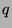sequence (the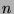th term of which is denoted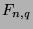) is defined recursively by the following statements:
•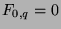.
•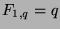.
•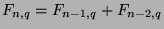,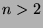.
Note that the regular Fibonacci numbers can be denoted by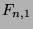in this notaion.
•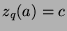means that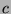has the following properties:
•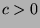.
•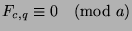.
•is the least positive integer satisfying these properties.
•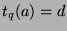means that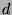has the following properties:
•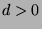.
•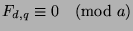.
•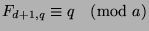.
•is the least positive integer satisfying these properties.
Note: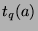is then the function that gives the period of the Fibonacci-sequence in modulo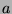.
•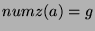means that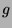has the following properties:
•is the number of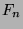that are congruent to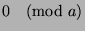, wheregoes from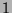to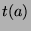, inclusive.
•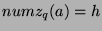means that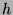has the following properties:
•is the number ofthat are congruent to, wheregoes fromto, inclusive.
•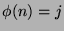(known as the Euler phi function) means that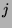has the following properties:
•is the number of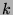such that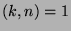, wheregoes fromto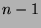, inclusive.Next: Theorems Up: Fibonacci-q numbers and new Previous: Fibonacci-q numbers and new   Contents
Gregory Stoll 2000-04-08